Mathematics is an equally important section for  REETMPTET & DSSSB Exams and has even more abundant importance in some other exams conducted by central or state govt. Generally, there are questions asked related to basic concepts and formulas of the Mensuration. To let you make the most of Mathematics section, we are providing important facts related to the Unit Digit. At least 2-3 questions are asked from this topic in most of the teaching exams. We wish you all the best of luck to come over the fear of the Mathematics section.

Mathematics Study Notes For All Teaching Exams

## Simplification

In simplification an expression, we must remove the brackets strictly in the order ( ), { }, [ ] and then we must apply the operations:

Of, Division, Multiplication, Addition and Subtraction.

‘BODMAS’ where B stands for bracket, O for of (‘Of’ means multiplication); D for division; M for Multiplication, A for Addition and S for Subtraction strictly in the order.

Division Algorithm:  Dividend = (Divisor × Quotient) + Remainder

Modulus or Absolute value : The absolute value of a real number X is denoted by the symbol |x| and is defined as –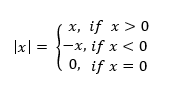How To Score 25+ Marks In Mathematics For CTET Exam?

Ex. : |5| = 5, |-5| = -(-5) = 5

In multiplication and division, when both the numbers carry similar sign, we get positive sign in the result otherwise we get negative sign in the result i.e.

(+) × (+)          = +

(+) × (-)           = –

(-) × (+)           = –

(-) × (-)           = +

(+) × (+)          = +

(+) × (-)           = –

(-) × (+)           = –

(-) × (-)           = +

Get free Study material for REET Exam

## Important terms:

Identity elements of Addition: ‘0’ (zero) is called identity element of addition of ‘O’ in any number does not affect that number.

e.g. x + 0 = x (x ∈ Q)

Identity element of Multiplication: ‘1’ is called identity element of multiplication as multiplication of ‘1’ in any number does not affect that number.

e.g. x × 1 = x

Inverse element of Addition / Negative element of Addition / Additive Inverse: The number is called “Additive inverse” of a certain number, when it is added to the certain number and result becomes ‘0’ (zero).

Ex.

• x + (-x) = 0

Here (-x) is Additive inverse of x.

• 9 + (-9) is Additive inverse of ‘9’

Inverse element of Multiplication / Reciprocal element / Multiplicative Inverse: The number is called “Multiplicative inverse” of a certain number, when the product of number and multiplication inverse is 1.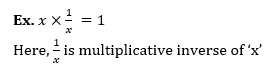How to Overcome Exam Fever, Especially When You Fear Maths

CONTINUED FRACTION: A continued fraction consists of the fractional denominators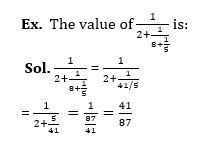Componendo and dividendo (C & D): It is a theorem on proportions that allows for a quick way to perform calculations and Reduce the amount of expansions needed It is particularly useful when dealing with equations involving fractions or rational functions.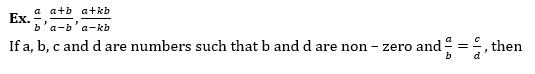Some Points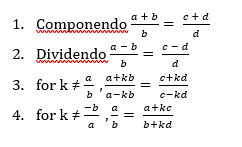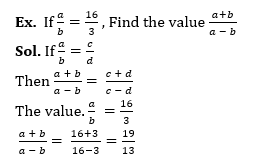Practice REET Previous Year Maths Quiz For REET Exam Here

## Recurring Number:

Pure recurring decimals: These are recurring decimals where the recurrence starts immediately after the decimal point.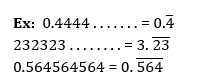Impure recurring decimals: Unlike pure recurring decimals, in these decimals, the recurrence occurs after a certain number of digits in the decimal.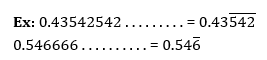•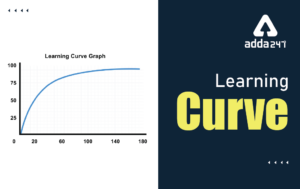Learning Curve - What is Learning Curve?...
•रस - परिभाषा, भे�...
•पठन कौशल- परिभा�...
•अलंकार - परिभाष�...
•Indian States and Capitals 2022: 28 Stat...
•New Education Policy(NEP) PDF - नई �...